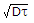# Electronics and Communication Engineering - Electronic Devices and Circuits - Discussion

50.

The minority carrier life time and diffusion constant in a semiconductor material are respectively 100 microsecond and 100 cm2/sec. The diffusion length is

 [A]. 0.1 cm [B]. 0.01 cm [C]. 0.0141 cm [D]. 1 cm

Answer: Option A

Explanation:

Diffusion length =.

 Thiru said: (Apr 9, 2014) Please explain using formula?

 Prakash said: (Aug 25, 2015) (100*100)/1000000 = 1/100. Under root of 1/100 1/10 = 0.1 cm.

 Saleem said: (Jul 23, 2017) I think the answer is 1cm.

 Sayan said: (Jul 29, 2017) L=(D.T)^1/2 [ D=The diffusion coefficient, L=diffusion length, T= specific life time). Here T=100 Sec = 10^(-4) sec. and D=100 cm^2/sec. Now L=(10^2/ 10^4)^(1/2)= (1/10) = 0.1 cm.

 Surekha said: (Aug 27, 2018) Explain in detail.

 Sean said: (Jan 21, 2021) √(100x10^-6 x 100)= 0.1cm.

#### Post your comments here:

Name *:

Email   : (optional)

» Your comments will be displayed only after manual approval.

#### Current Affairs 2021

Interview Questions and Answers